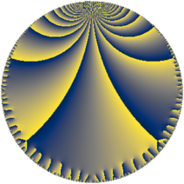# Properties

 Label 38.7.fLevel $38$ Weight $7$ Character orbit 38.f Rep. character $\chi_{38}(3,\cdot)$ Character field $\Q(\zeta_{18})$ Dimension $60$ Newform subspaces $1$ Sturm bound $35$ Trace bound $0$

# Related objects

## Defining parameters

 Level: $$N$$ $$=$$ $$38 = 2 \cdot 19$$ Weight: $$k$$ $$=$$ $$7$$ Character orbit: $$[\chi]$$ $$=$$ 38.f (of order $$18$$ and degree $$6$$) Character conductor: $$\operatorname{cond}(\chi)$$ $$=$$ $$19$$ Character field: $$\Q(\zeta_{18})$$ Newform subspaces: $$1$$ Sturm bound: $$35$$ Trace bound: $$0$$

## Dimensions

The following table gives the dimensions of various subspaces of $$M_{7}(38, [\chi])$$.

Total New Old
Modular forms 192 60 132
Cusp forms 168 60 108
Eisenstein series 24 0 24

## Trace form

 $$60q + 30q^{3} - 240q^{6} + 432q^{7} + 690q^{9} + O(q^{10})$$ $$60q + 30q^{3} - 240q^{6} + 432q^{7} + 690q^{9} - 3360q^{11} + 9660q^{13} + 2496q^{14} - 37464q^{15} + 7704q^{17} + 46704q^{19} + 21504q^{20} + 78732q^{21} - 20304q^{22} - 54120q^{23} + 7680q^{24} - 41328q^{25} - 25536q^{26} - 124830q^{27} + 65280q^{28} - 21840q^{29} + 30780q^{31} - 54582q^{33} - 70560q^{34} - 56796q^{35} - 22080q^{36} + 52080q^{38} + 496344q^{39} + 83394q^{41} + 38880q^{42} - 294384q^{43} - 8448q^{44} - 391824q^{45} + 738720q^{46} + 639264q^{47} + 61440q^{48} - 85530q^{49} - 689472q^{50} - 215478q^{51} - 456960q^{52} - 1189428q^{53} - 907200q^{54} - 316512q^{55} + 517776q^{57} + 440640q^{58} + 957390q^{59} + 586752q^{60} + 1390404q^{61} + 978384q^{62} + 2002152q^{63} + 983040q^{64} - 878940q^{65} - 976992q^{66} - 2920854q^{67} - 78144q^{68} - 2667600q^{69} - 643392q^{70} + 1585980q^{71} + 706560q^{72} + 1734648q^{73} - 1167552q^{74} - 411264q^{76} - 1481400q^{77} - 2170608q^{78} + 4991952q^{79} + 40002q^{81} + 2299392q^{82} - 30672q^{83} + 1313280q^{84} + 815976q^{85} - 1424976q^{86} - 125892q^{87} - 6804636q^{89} - 2283456q^{90} - 3838704q^{91} - 244992q^{92} - 3271128q^{93} - 4631640q^{95} + 2112390q^{97} + 7177728q^{98} + 21467982q^{99} + O(q^{100})$$

## Decomposition of $$S_{7}^{\mathrm{new}}(38, [\chi])$$ into newform subspaces

Label Dim. $$A$$ Field CM Traces $q$-expansion
$$a_2$$ $$a_3$$ $$a_5$$ $$a_7$$
38.7.f.a $$60$$ $$8.742$$ None $$0$$ $$30$$ $$0$$ $$432$$

## Decomposition of $$S_{7}^{\mathrm{old}}(38, [\chi])$$ into lower level spaces

$$S_{7}^{\mathrm{old}}(38, [\chi]) \cong$$ $$S_{7}^{\mathrm{new}}(19, [\chi])$$$$^{\oplus 2}$$